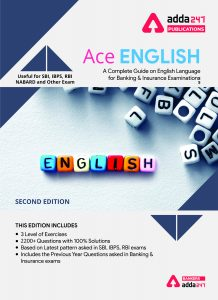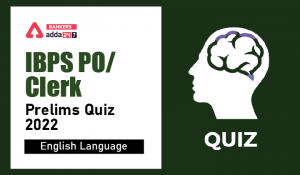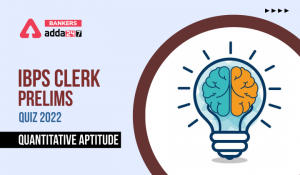Latest Banking jobs   »

# English Quizzes For IBPS Clerk Prelims 2022- 18th July

Directions (1-10): Read each sentence to find out whether there is any grammatical error or idiomatic error in it. The error, if any, will be in one part of the sentence. The number of that part is the answer. If there is no error, the answer is (e). (Ignore errors of punctuation, if any.)

Q1. I began to suspect (a)/ trouble as soon as (b)/ one of the boys (c)/ threw a stone at the policemen. (d)/ No error. (e)
(a) A
(b) B
(c) C
(d) D
(e) E

Q2. It is all the more better (a)/ for you to take (b)/ the examination than to (c)/ withdraw your form. (d)/ No error. (e)
(a) A
(b) B
(c) C
(d) D
(e) E

Q3. When a group of teenagers visited (a)/ the entertainment centre (b)/ little did they know (c)/ that their outing will lead them to a hospital.(d)/ No error. (e)
(a) A
(b) B
(c) C
(d) D
(e) E

Q4. If you had not been (a)/ so secretive you had (b)/ told me all the facts earlier (c)/ I could have helped you. (d)/ No error. (e)
(a) A
(b) B
(c) C
(d) D
(e) E

Q5. Although there are some similarities (a)/ in the qualification of both the (b)/ candidates the differences among them (c)/ are considerably pronounced. (d)/ No error. (e)
(a) A
(b) B
(c) C
(d) D
(e) E

Q6. The king did not mind (a)/ for putting the old people (b)/ to death because he (c)/ was young himself. (d)/ No error. (e)
(a) A
(b) B
(c) C
(d) D
(e) E

Q7. If I were you (a)/ I would not (b)/ attend the (c)/ function. (d)/ No error. (e)
(a) A
(b) B
(c) C
(d) D
(e) E

Q8. It is high time (a)/ he stood on (b)/ his (c)/ own two legs. (d)/ No error. (e)
(a) A
(b) B
(c) C
(d) D
(e) E

Q9. Knowledge of (a)/ at least two languages (b)/ are required to (c)/ pass the examination. (d)/ No error. (e)
(a) A
(b) B
(c) C
(d) D
(e) E

Q10. When I arrived (a)/ I found them both (b)/ sitting on the chair (c)/ and waiting for me. (d)/ No error. (e)

(a) A
(b) B
(c) C
(d) D
(e) E

Directions (11-15): Which of the following phrases (I), (II), and (III) given below each sentence should replace the phrase printed in bold letters to make the sentence grammatically correct? Choose the best option among the five given alternatives that reflect the correct use of phrase in the context of the grammatically correct sentence. If the sentence is correct as it is, mark (e) i.e., “No correction required” as the answer.

Q11. Had I realised your house was such a long way off I would take a taxi.
(I) would have taken a taxi
(II) may had taken a taxi
(III) could have taken a taxi

(a)Only (I) is correct
(b)Only (III) is correct
(c)Both (I) and (II) are correct
(d)Both (I) and (III) are correct
(e)No correction required

Q12. Scarcely we had reached the office when it started raining cats and dogs.

(a)Only (I) is correct
(b)Only (III) is correct
(c)Both (I) and (II) are correct
(d)Both (II) and (III) are correct
(e)No correction required

Q13. The perquisites attached to this job makes it even more attractive than the salary indicated.

(I) with this job makes it
(II) to this job made it
(III)to this job make it

(a)Only (I) is correct
(b)Only (III) is correct
(c)Both (I) and (II) are correct
(d)Both (II) and (III) are correct
(e)No correction required

Q14. I don’t care as you invite.

(I) whom you invite
(II) who you invite
(III)whom should invite.

(a)Only (I) is correct
(b)Only (III) is correct
(c)Both (I) and (II) are correct
(d)Both (II) and (III) are correct
(e)No correction required

Q15. The book is well illustrated and attractively bound making altogether an attractive volume.

(I) that make altogether
(II) which makes altogether an
(III)making it altogether an

(a)Only (I) is correct
(b)Only (III) is correct
(c)Both (I) and (II) are correct
(d)Both (II) and (III) are correct
(e)No correction required

Solutions

S1. Ans. (e)
Sol. The sentence is grammatically correct.

S2. Ans. (a)
Sol. The use of ‘more’ is superfluous because ‘better’ itself is a comparative degree.

S3. Ans. (d)
Sol. ‘would’ will be used in place of ‘will’ as the sentence is in past tense.

S4. Ans. (b)
Sol. Use ‘and’ before ‘you’ as there are two clauses in the conditional clauses of the sentences which should be connected with ‘and’.

S5. Ans. (c)
Sol. ‘between’ will be used in place of ‘among’ as ‘between’ is used for two things or person.

S6. Ans. (b)
Sol. ‘for’ will not be used as after ‘mind, propose, suggest, admit, avoid, fancy, enjoy’, V4 (V+ ing) is used and this ‘V4’ is used as gerund.
Ex. I avoid meeting politicians.

S7. Ans. (e)
Sol. The sentence is grammatically correct.

S8. Ans. (d)
Sol. The use of ‘two’ is superfluous.

S9. Ans. (c)
Sol. ‘is’ will be used in place of ‘are’ as the subject of the sentence ‘knowledge’ is singular.

S10. Ans. (e)
Sol. The sentence is grammatically correct.

S11. Ans. (d)
Sol. ‘would have taken’ will be used in place of ‘would take’ as ‘had+ subject+ V3… subject+ would+ have+ V3’ is used. (III) is also correct in the same way.
Ex. Had he worked hard, he would have succeeded.

S12. Ans. (c)
Sol. ‘had we’ will be used in place of ‘we had’ as verb is used before the subject with ‘scarcely’ or ‘hardly’. (I) and (II) both are correct.
No sooner takes ‘than’ with it but in the given statement ‘when’ is used. Therefore (III) is incorrect.

S13. Ans. (d)
Sol. ‘make’ will be used in place of ‘makes’ as the subject of the sentence ‘perquisites’ is plural. Therefore (III) is correct.
(II) is also correct.

S14. Ans. (a)
Sol. ‘who’ should be replaced by ‘whom’

S15. Ans. (b)
Sol. Pronoun ‘it’ will be used in the form of object after ‘making’ for ‘book’.#### Congratulations!Incorrect details? Fill the form again here

•English Quizzes For IBPS Clerk/PO Prelim...
•English Quizzes For IBPS Clerk/PO Prelim...
•Quantitative Aptitude Quiz For IBPS Cler...
•Quantitative Aptitude Quiz For IBPS Cler...
•Reasoning Ability Quiz For IBPS Clerk/PO...
•Quantitative Aptitude Quiz For IBPS Cler...